# How to Use Python for Learning Vector Quantization From ScratchLearning vector quantization (LVQ) is a prototype-based learning method. A supervised learning classification algorithm, it can be used as an alternative to some machine learning (ML) algorithms. While the actual algorithm isn't especially strong, it is simple and instinctive. It also has a few expansions that make it a powerful tool in various ML-related works. In this article, we will explore learning vector quantization in detail and how to implement it in Python.

## How does learning vector quantization work?

Learning vector quantization is similar to self-organizing maps. At least one prototype is used to address each class in the dataset. Every prototype is depicted as a point in the feature space. New (obscure) data points are then allotted the class of the prototype that is closest to them. To select the closest point, a distance measure must be characterized. The Euclidean distance metric is a good choice to calculate the next closest point.

There is no constraint on the number of prototypes that can be utilized per class. However, there should be at least one prototype for each class. The picture beneath shows a straightforward learning vector quantization framework where each class (red and green) is addressed by a prototype.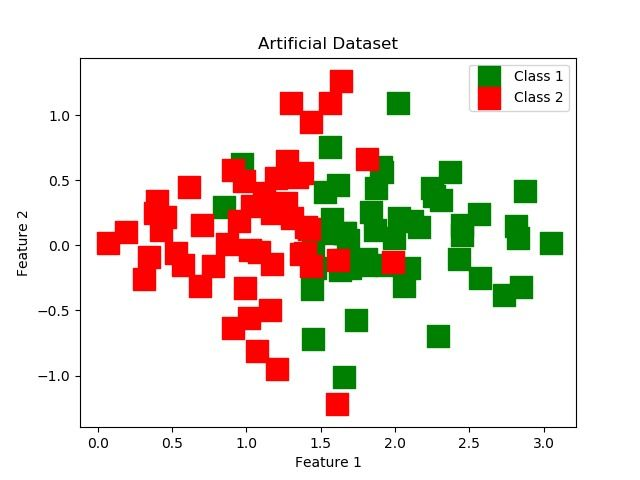Image source: Medium.com

How do we fit prototypes to each class so that they are a decent portrayal of that class? We start by choosing a distance metric. In this model, we will apply the Euclidean distance metric.

Note that the Euclidean distance between two vectors in N dimensions is given by: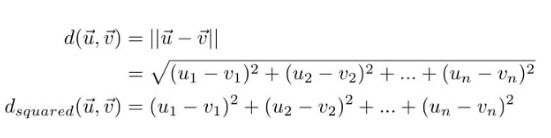We can utilize the squared Euclidean distance which doesn't expect us to figure the square root.

## The architecture of learning vector quantization

The basic architecture of learning vector quantization consists of two layers: the input layer and the output layer. The image below shows the structure of the algorithm.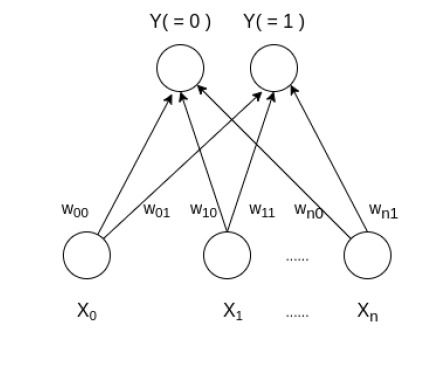Image source: GeeksforGeeks

Here, we have ‘n’ number of input units and ‘m’ number of output units. The layers are interconnected to each other by having weights on them.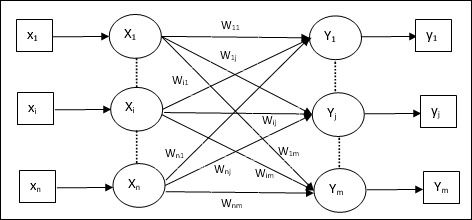Image source: CentOS

## The math behind learning vector quantization

Let’s take a look at the mathematical concept behind LVQ.

Consider the following five input vectors and their target class.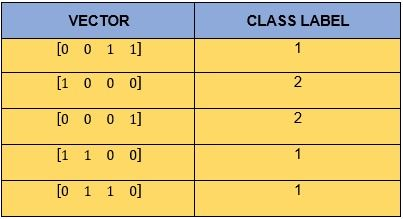In each input vector, there are four input components (x1, x2, x3, x4) and two target classes (1, 2).

Let’s assign the weights based on the class. As there are two target classes, the first two vectors can be used as weight vectors as w1 = [ 0 0 1 1 ] & w2 = [ 1 0 0 0 ].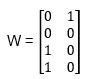The remaining three vectors can be used for training.

Consider the learning rate 𝛼 as 0.1.

Let’s take our first input vector (the third vector).

Input vector: [ 0 0 0 1 ]

Target class: 2

The next step is to calculate the Euclidean distance. The formula is: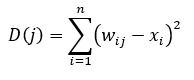where,

wij is the weight

xi is the input vector component.

Now, we can calculate D(1) and D(2) which are the distance of the input unit from the first and second weight vectors, respectively.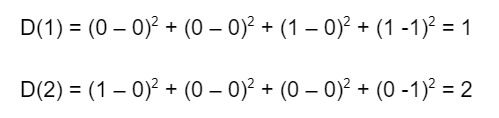Here, D(1) is lesser than D(2) and the winner index is J = 1.

Since the target class 2 is not equal to J, updating the weight can be done by: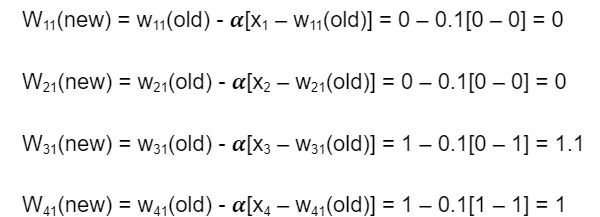The updated weight vector will be: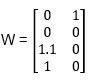Let’s repeat the same process for the rest of the input vectors.

Input vector: [ 1 1 0 0 ]

Target class: 1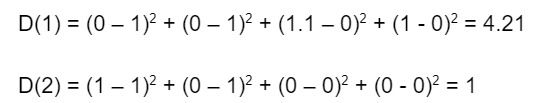Here, D(2) is less than D(1) and the winner index is J = 2.

Since the target class 2 is not equal to J, updating the weight can be done by: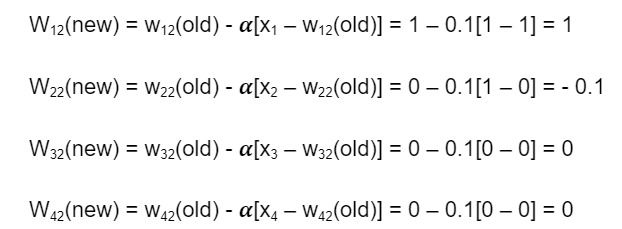The updated weight vector will be: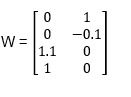Input vector: [ 0 1 1 0 ]

Target class: 1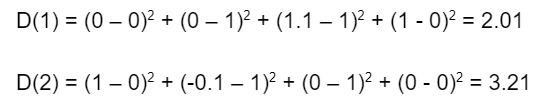Here, D(1) is lesser than D(2) and the winner index is J = 1.

Since the target class 2 is equal to J, updating the weight can be done by: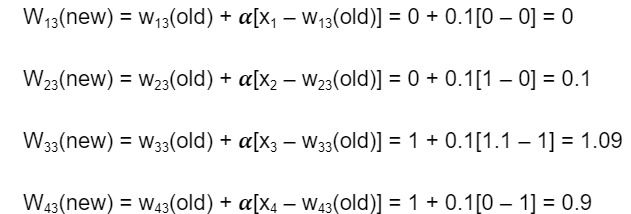The updated weight vector will be: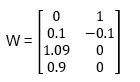This is the end of the first iteration, i.e., an epoch. We have applied weights on all three input vectors. Similarly, we can perform ‘n’ number of epochs till all the winning vectors become equal to the target class of the input vectors.

## Algorithm of learning vector quantization

Let’s take a look at a simplified view of the LVQ algorithm.

STEP 1

Initialize the weights.

STEP 2

Select the training sample for the given number of epochs.

STEP 3

Calculate the feature vector and update it.

STEP 4

Repeat the steps for all the training samples.

STEP 5

Predict the test examples.

## How to implement learning vector quantization in Python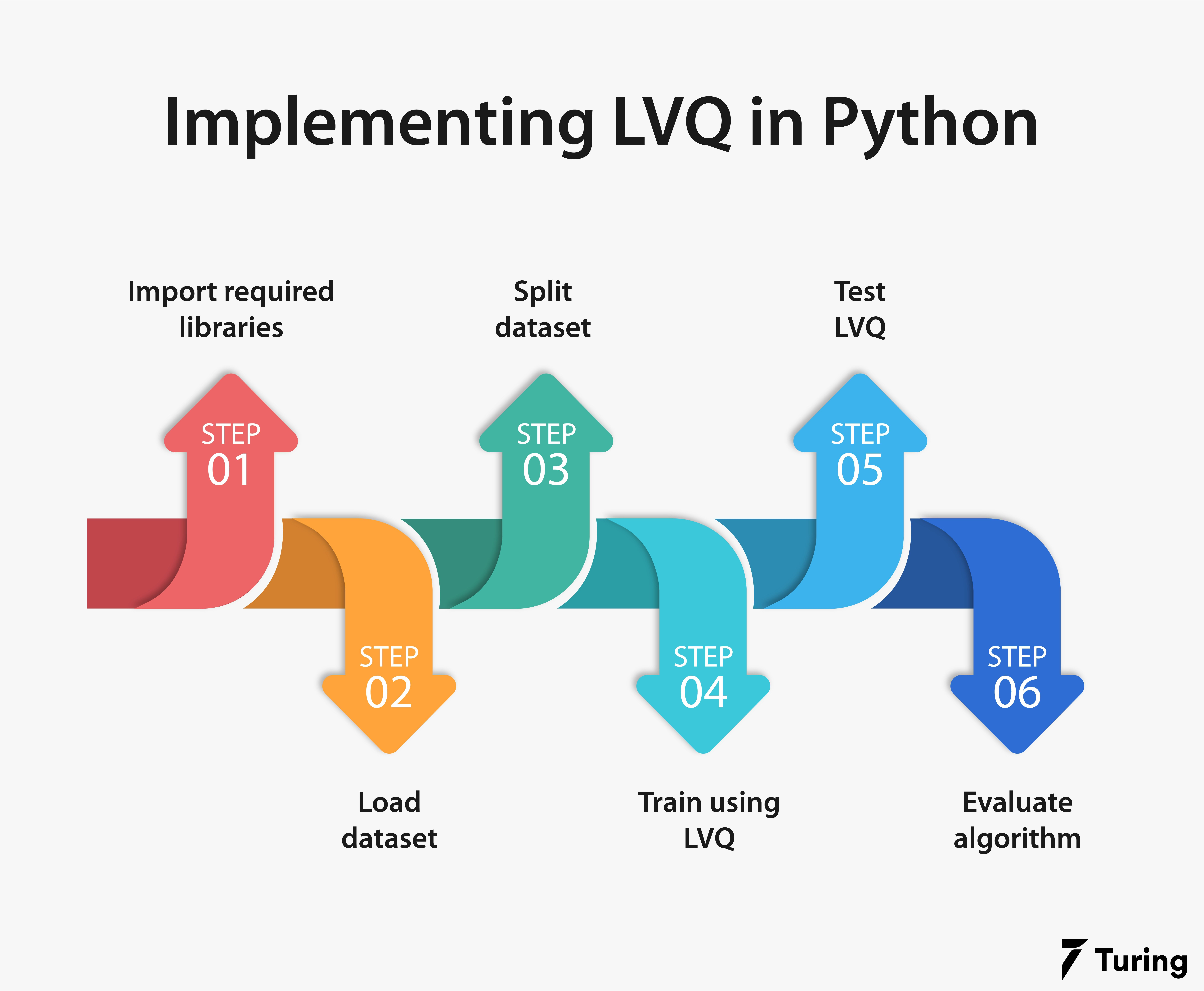Below is the Python code to implement LVQ.

In this example, we will use the digits dataset available in sklearn. It contains 1797 images, each of which is 8x8 pixels.

### Import the necessary libraries

The first step is to import the required libraries.

``````from sklearn import datasets
from sklearn.model_selection import train_test_split
from sklearn.preprocessing import minmax_scale
from sklearn.metrics import precision_score, recall_score, accuracy_score, f1_score
import matplotlib.pyplot as plt
import numpy as np
import math

``````

We then load the digits dataset and print it.

``````digits = datasets.load_digits()
digits

``````

Output: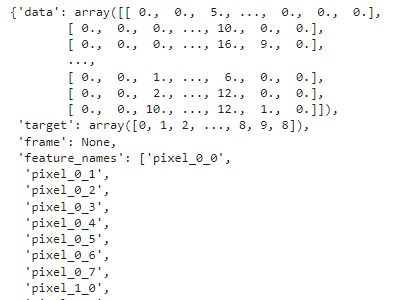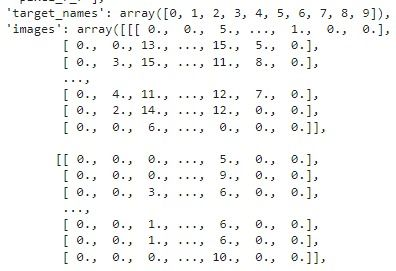We print the input image.

``````plt.imshow(digits.images[-2], cmap='gray_r')
plt.show()

``````

Output: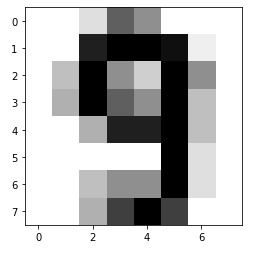We can check the image by printing the target.

``````digits.target[-2]

``````

Output: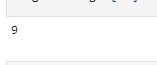### Split the dataset

The next step is to split the dataset into training and testing data.

``````X = digits.data
Y = digits.target
X, Y

``````

Output: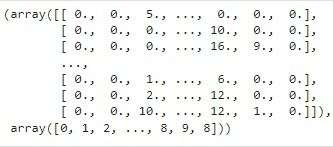``````x_train, x_test, y_train, y_test = train_test_split(X, Y, shuffle=False, test_size=0.3)

``````

### Train using LVQ

Next, we define the training phase of the data using the LVQ algorithm.

``````def lvq_train(X, y, a, b, max_ep, min_a, e):
c, train_idx = np.unique(y, True)
r = c
W = X[train_idx].astype(np.float64)
train = np.array([e for i, e in enumerate(zip(X, y)) if i not in train_idx])
X = train[:, 0]
y = train[:, 1]
ep = 0

while ep < max_ep and a > min_a:
for i, x in enumerate(X):
d = [math.sqrt(sum((w - x) ** 2)) for w in W]
min_1 = np.argmin(d)

min_2 = 0
dc = float(np.amin(d))
dr = 0
min_2 = d.index(sorted(d))
dr = float(d[min_2])
if c[min_1] == y[i] and c[min_1] != r[min_2]:
W[min_1] = W[min_1] + a * (x - W[min_1])

elif c[min_1] != r[min_2] and y[i] == r[min_2]:
if dc != 0 and dr != 0:

if min((dc/dr),(dr/dc)) > (1-e) / (1+e):
W[min_1] = W[min_1] - a * (x - W[min_1])
W[min_2] = W[min_2] + a * (x - W[min_2])
elif c[min_1] == r[min_2] and y[i] == r[min_2]:
W[min_1] = W[min_1] + e * a * (x - W[min_1])
W[min_2] = W[min_2] + e * a * (x- W[min_2])
a = a * b
ep += 1
return W, c

``````

### Test the LVQ

We then define the testing function for the data.

``````def lvq_test(x, W):

W, c = W
d = [math.sqrt(sum((w - x) ** 2)) for w in W]

return c[np.argmin(d)]

``````

### Evaluate the algorithm

We start training the data.

``````W = lvq_train(x_train, y_train, 0.2, 0.5, 100, 0.001, 0.3)
W

``````

Output: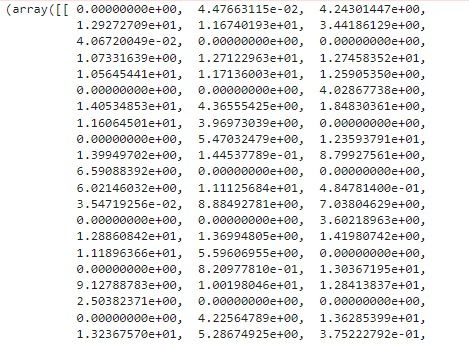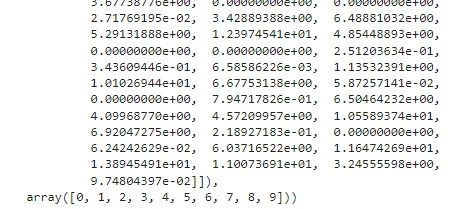We test the algorithm.

``````predicted = []
for i in x_test:
predicted.append(lvq_test(i, W))

``````

We have now completed the training and testing of the data.

Let’s evaluate our model and check the accuracy.

``````def print_metrics(labels, preds):
print("Precision Score: {}".format(precision_score(labels,
preds, average = 'weighted')))
print("Recall Score: {}".format(recall_score(labels, preds,
average = 'weighted')))
print("Accuracy Score: {}".format(accuracy_score(labels,
preds)))
print("F1 Score: {}".format(f1_score(labels, preds, average =
'weighted')))
print_metrics(y_test, predicted)

``````

Output: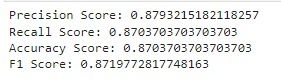Code source

The accuracy is 87% which is a decent score.

Go ahead and implement LVQ with a dataset of your choosing. You can also improve the algorithm. For example, by taking into consideration various prototypes to be utilized per class.

## Variants of learning vector quantization

The LVQ algorithm has other variants, namely LVQ2, LVQ2.1, and LVQ3. They were developed by Teuvo Kohonen.

### LVQ2 algorithm

The second improved version of the LVQ algorithm is similar to Bayesian decision theory.

The steps for LVQ2 are the same as for LVQ. However, there are a few differences. In the LVQ2 algorithm, the weights are applied in certain conditions such as:

• When the input vector is incorrectly classified.
• When the next closest vector classifies correctly.
• When the input vector is enough to get the decision boundary.

Here, learning takes place only when the input vector comes within a window which can be updated as follows.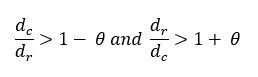Updating the weights can be done by: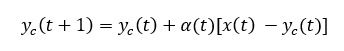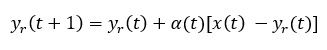where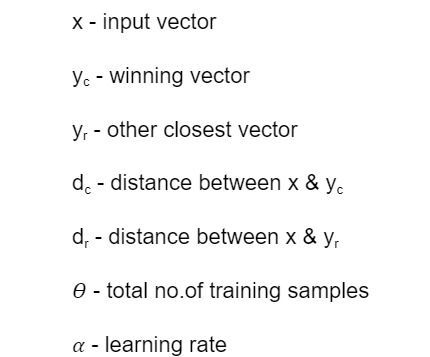### LVQ2.1 algorithm

LVQ2.1 is a popular variant of learning vector quantization. In LVQ2, the weights are updated at two conditions: one for the winning vector which has the same class label, and the other for the next vector which has a different class label. However, in LVQ2.1, either vector may have the same class labels.

Here, the condition for the window within which the input vector fits can be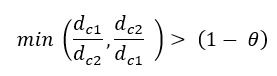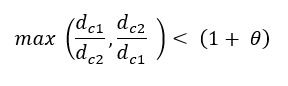The weights can be updated by: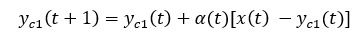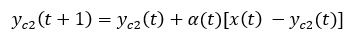### LVQ3 algorithm

In LVQ3, learning is further extended for the cases where the input vector, the winning vector, and the other closest vector belong to the same class label.

Here, the window condition can be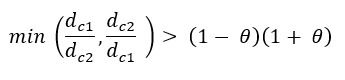The weights can be updated by: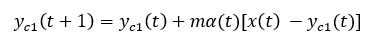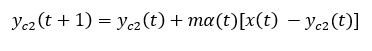where m ranges from 0.1 < m < 0.5 is a stabilizing constant.

## Pros and cons of learning vector quantization

LVQ offers several benefits. It is straightforward, instinctive, and simple to execute while yielding respectable performance. It can be considered one of the most powerful algorithms for prototype-based classification. Even though other popular ML algorithms such as support vector machines and other deep learning architectures can achieve excellent results, LVQ is a smart alternative. It enables lower complexity and reduces computational costs.

Note, however, that the Euclidean distance can bring about issues if the data has many aspects or is noisy. Appropriate standardization and preprocessing of features are necessary. Dimensionality reduction also needs to be performed if the dataset has many dimensions.

## Real-world applications of learning vector quantization

LVQ is mostly used in:

• Fraud detection
• Intelligent sensor systems
• Real-time adaptive traffic signal control
• Fault diagnosis
• Text categorization
• Multi-class classification.

In this article, we learnt the basics of the learning vector quantization algorithm, its architecture, and its workflow. We also implemented it using Python. Practice it using various datasets to get a better understanding of how it works.

## Author

•### Turing

Author is a seasoned writer with a reputation for crafting highly engaging, well-researched, and useful content that is widely read by many of today's skilled programmers and developers.

Learning vector quantization is a supervised learning algorithm that is mainly used for classification problems.

Learning vector quantization is a supervised learning algorithm for classifying input data using simple vector and distance calculations. Vector quantization is an unsupervised destiny estimator.

LVQ is related to the KNN algorithm. One of the disadvantages of KNN is that the entire training dataset needs to be analyzed. On the other hand, LVQ allows you to select the number of training samples of your choice. It reduces memory utilization.

One of the important advantages of learning vector quantization is the predefined model complexity that is determined by the prototypes. It uses limited memory and offers faster calculations.### Press

What's up with Turing? Get the latest news about us here.### Blog